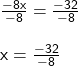## Instructions: Solve the following linear equation. – 2x + 38 = 2(3 + 3x) 2-

Question

Instructions: Solve the following linear
equation.
– 2x + 38 = 2(3 + 3x)
2-

in progress 0
6 months 2021-08-09T07:19:32+00:00 2 Answers 1 views 0

x = 4

Step-by-step explanation:

• -2x + 38 = 2(3 + 3x)

Use distributive property to multiply 2 by 3 and 3x

• -2x + 38 = 2 ×3 + 2 × 3x
• -2x + 38 = 6 + 6x

subtract 6x from both side

• -2x + 38 – 6x = 6 + 6x – 6x

combine -2x and -6x to get -8x

• -8x + 38 = 6

subtract 38 from both side

• -8x + 38 – 38 = 6 – 38

subtract 38 from 6 to get -32

• -8x = -32

divide both side by -8divide -32 by -8 to get 4

• x = 4

Step-by-step explanation:

-2x +38 = 2(3 + 3x)

-2x + 38 = 2*3 + 2*3x

-2x + 38 = 6 + 6x

38 = 6 + 6x + 2x

Combine like terms

38 = 6 + 8x

Subtract 8  from both sides

38 – 6 = 8x

8x = 32

Divide both sides by 8

x = 32/8

x = 4